# A series RLC circuit

A series `RLC` circuit consists of a resistor, a capacitor, and an inductor connected in series, as shown in figure 3.36. If `R` , `L` , and `C` are the resistance, inductance, and capacitance, then the relations between voltage ( `v` ) and current ( `i` ) for the three components are described by the equations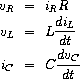and the circuit connections dictate the relations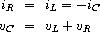Combining these equations shows that the state of the circuit (summarized by `vC` , the voltage across the capacitor, and `iL` , the current in the inductor) is described by the pair of differential equations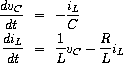The signal-flow diagram representing this system of differential equations is shown in figure 3.37.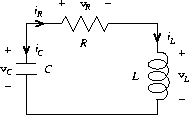Figure 3.36: A series RLC circuit.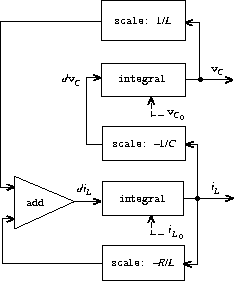Figure 3.37: A signal-flow diagram for the solution to a series RLC circuit.

Write a procedure `RLC` that takes as arguments the parameters `R` , `L` , and `C` of the circuit and the time increment `dt` . In a manner similar to that of the `RC` procedure of exercise 3.73 , `RLC` should produce a procedure that takes the initial values of the state variables, `vC₀` and `iL₀` , and produces a pair (using `cons` ) of the streams of states `vC` and `iL` . Using `RLC` , generate the pair of streams that models the behavior of a series `RLC` circuit with `K = 1` ohm, `C = 0.2` farad, `L = 1` henry, `dt = 0.1` second, and initial values `iL₀ = 0` amps and `vC₀ = 10` volts.